# Magic number

The number 135 split to two addends so that one addend was 30 greater than 2/5 the addend.

a =  60
b =  75

### Step-by-step explanation:

a+b=135
a = 30 + 2/5 b

a+b=135
a = 30 + 2/5•b

a+b = 135
5a-2b = 150

a = 60
b = 75

Our linear equations calculator calculates it.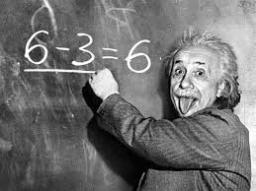Did you find an error or inaccuracy? Feel free to write us. Thank you!Tips to related online calculators
Do you have a system of equations and looking for calculator system of linear equations?

## Related math problems and questions:Number 118 divide into two addends, so the first addend is 69 greater than 75% of the second addend.Divide number 135 into two additions so that one adds 30 more than 2/5 of the other add. Write the bigger one.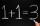Number 839 divide into the two addends that the first was 17 greater than 60% of the second. Determine these addends.
• Divisible by 5How many three-digit odd numbers divisible by 5, which are in place ten's number 3?
• Reminder and quotientThere are given numbers A = 135, B = 315. Find the smallest natural number R greater than 1 so that the proportions R:A, R:B are with the remainder 1.
• Resort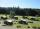In a completely crowded resort was 30% from the Czech Republic quarter visitors were from Slovakia and the rest 135 came from Germany. What capacity have resort?
• Two workers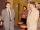Two workers make 138 parts/items. The first one produces 30% more than the second. How many items will each produce?
• Ratio of two unknown numbersTwo numbers are given. Their sum is 30. We calculate one-sixth of a larger number and add to both numbers. So we get new numbers whose ratio is 5:7. Which two numbers were given?
• Area 4gonCalculate the area of 4-gon, two, and the two sides are equal and parallel with lengths 11, 5, 11, and 5. Inner angles are 45°, 135°,45°, 135°.
• Classs meanClass size is 30 students, math was not worse than sign 2. Determine the number of pupils who were sign 1 if the class had a 1.4 sign average.
• Unknown number 10Number first increased by 30%, then by 1/5. What percentage we've increased the original number?
• Three numbersFind three numbers so that the second number is 4 times greater than the first and the third is lower by 5 than the second number. Their sum is 67.
• Logistics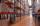Head of logistics department informed the meeting of management of the same item is currently not available in sufficient quantity in all three warehouses of the company. We have the total 135 pieces. It was 37 pieces more in the warehouse No. 3 than in t
• MotionFrom two different locations distant 232 km started against car and bus. The car started at 5:20 with average speed 64 km/h. Bus started at 7:30 with average speed 80 km/h. When they meet? How many kilometers went the bus?
• Pair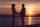They have together 39 years - he has 2 times as she was when he was equal as she has today ....
• Three siblings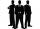Three siblings have birthday in one day-today. Together they have 35 years today. The youngest is three years younger than middle and the oldest is 5 years older than middle. How old is each?
• Unknown mixed numberFind the number that is smaller than 5 5/12 by as much as 2 2/13 is smaller than 6 1/6